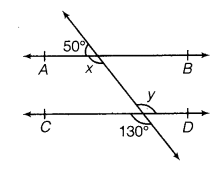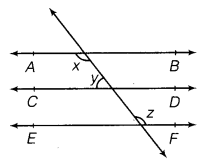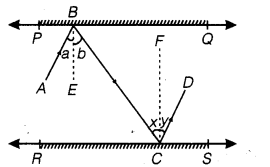# CLASS 9 MATH NCERT SOLUTION FOR CHAPTER – 6 LINES AND ANGLES EX – 6.2

## Lines and Angles

Question 1.
In the given figure, find the values of x and y and then show that  AB||CD.Solution:
Here, l is a straight line,
So, x + 50° = 180° [by linear pair axiom]
⇒ x = 180° – 50° = 130°
Now, line l and CD intersect each other at a point.
So,          y=130°                       [vertically opposite angles]
Thus,     x = y = 130°               [alternate interior angles]
Hence,   AB || CD                     [by theorem 2] Hence proved.

Question 2.
In the given figure, if AB || CD, CD || EF and y :z = 3: 7, then find the value of x.Solution:
Given, AB || CD and CD || EF
∴      AB ||EF
[since, lines which are parallel to the same line, are parallel to each other]
Then,   ∠x= ∠z              [alternate interior angles] … (i)
Also, given that y : z = 3 :7
⇒ y : x =3:7                                 [from eq. (i)] …(ii)
Let ∠y = 3a and ∠x=7a
Now, AB || CD
∠x + ∠y= 180° [since, interior angles are supplementary]
⇒ 7a +3a = 180°⇒ 10a = 180° ⇒ a =$\cfrac { 180 }{ 10 }$  = 18°
Hence, x = 7a = (7 x 18)° = 126°

Question 3.
In the given figure, if AB || CD, FE ⊥ CD, and ∠GED = 126°, find ∠AGE, ∠GEF and ∠FGE.Solution:
Given, AB || CD, EF ⊥ CD and GE is a transversal.
.’.    ∠AGE = ∠GED [alternate interior angles]
∠AGE = 126° [∠AGE =126° given]
∠GEF = ∠GED – ∠FED
⇒ ∠GEF = 126° – 90° [∵  FE ⊥ CD => FED = 90°]
⇒ ∠GEF= 36°
Also, AB is a straight line and EG is a ray on it.
So, ∠FGE + ∠AGE = 180° [By linear pair axiom)
⇒  ∠FGE +126° = 180° [ ∵ ∠AGE= 126°]
⇒  ∠FGE = 180° -126° = 54°
Hence, ∠AGE = 126°, ∠GEF = 36° and ∠FGE =54°.

Question 4.
In the given figure, if PQ || ST, ∠PQR = 110° and ∠RST = 130°, find ∠QRS.
[Hint: Draw a line parallel to ST through point R.]Solution:

Given, PQ || ST, ∠PQR = 110° and ∠RST = 130°Draw a line AB parallel to ST through R.
Now, ST || AB and SR is a transversal.
So, ∠RST + ∠SRB = 180°[since, sum of the interior angles on the same side of the transversal is 180°]
⇒ 130° + ∠SRB = 180° ⇒ ∠SRB = 180°-130°
⇒  ∠SRB = 50°    …(i)
Since, PQ || ST and AB || ST, so PQ || AB and then QR is a transversal.
So, ∠PQR + ∠QRA = 180° [since, sum of the interior angles on the same side of the transversal is 180°] ⇒ 110° + ∠QRA = 180° ⇒ ∠QRA = 180° -110°
⇒ ∠QRA=70°  ..(ii)
Now, ARB is a line.
∴  ∠QRA + ∠QRS + ∠SRB = 180° [by linear pair axiom]
⇒ = 70° + ∠QRS + 50° = 180° ⇒ 120° + ∠QRS = 180°
⇒ => ∠QRS = 180° -120° ⇒ ∠QRS = 60°

Question 5.
In the given figure, if AB||CD, ∠APQ 50° and ∠PRD = 127°, find x and y.

Solution:

Given, ∠APQ = 50° and ∠PRD = 127°
In the given figure,
AB || CD and PQ is a transversal.
∴ ∠APQ + ∠PQC = 180°
[Since, pair of consecutive interior angles on the same side of the transversal is 180°]
⇒ 50° + ∠PQC = 180°  [∴ ∠APQ = 50°, given]
⇒ ∠PQC = 180° -50° = 130°
Now, CD is a straight line and QP is a ray on it
So, ∠PQC + ∠PQR = 180° [by linear pair axiom]
⇒ 130° + ∠PQR = 180°   [∴ ∠PQC = 130°]
⇒  ∠PQR = 180° -130° = 50°
∴  x = 50°    [∴ ∠PQR = x]
Also, AB || CD and PR is a transversal.
So, ∠APR = ∠PRD  [alternate interior angles]
⇒ 50° + y = 127°  [∴ ∠APR = ∠APQ + ∠QPR = 50°+y]
⇒ y = 127° – 50°  ⇒ y=77°
Hence, x = 50° and y = 77°.

Question 6.
In the given figure, PQ and RS are two mirrors placed parallel to each other. An incident ray AB strikes the mirror PQ at B, the reflected ray moves along the path BC and strikes the mirror RS at C and again reflects back along CD. Prove that
AB || CD.Solution:
Draw BE ⊥ PQ and CF ⊥ L RS.
⇒ BE || CF
Also,  ∠a= ∠b      …(i)  [∵ angle of incidence = angle of reflection]
and    ∠x= ∠y   …(ii) [∵ angle of incidence = angle of reflection]Since, BE ||CF and BC is transversal.
∴ ∠b= ∠x    [alternate interior angles]
⇒ 2∠b = 2∠x   [multiplying by 2 on both sides]
⇒ ∠b + ∠b = ∠x + ∠x
⇒ ∠a + ∠b = ∠x + ∠y                    [from eqs. (i) and (ii)]
⇒  ∠ABC = ∠DCB
which are alternate interior angles.
Hence, AB || BC Hence proved.